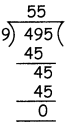Tamilnadu State Board New Syllabus Samacheer Kalvi 4th Maths Guide Pdf Term 3 Chapter 2 Numbers Ex 2.1 Textbook Questions and Answers, Notes.

## Tamilnadu Samacheer Kalvi 4th Maths Solutions Term 3 Chapter 2 Numbers Ex 2.1

Simplify the following

Question 1.
896 ÷ 5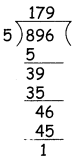Question = 179
Remainder = 1Question 2.
696 ÷ 6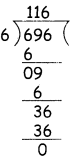Question = 116
Remainder = 0

Question 3.
686 ÷ 7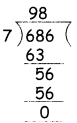Question = 98
Remainder = 0Question 4.
813 ÷ 8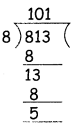Question = 101
Remainder = 5

Question 5.
891 ÷ 8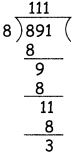Question = 111
Remainder = 3Question 6.
703 ÷ 2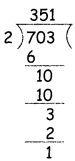Question = 351
Remainder = 1

Question 7.
Rahul has 192 toy cars. He put them equally in 6 boxes. How many toy cars will he put in each box? How many toy cars are left over?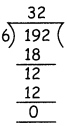Question = 32
Remainder = 0
32 toys in each box. No toys left over.Question 8.
Akila has 495 photographs to put in an album. She can put 9 photographs on each page. How many pages can she fill?